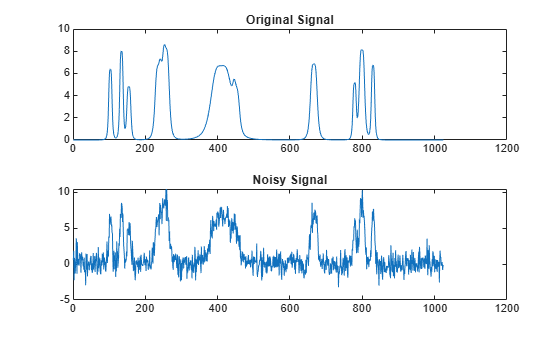## Translation Invariant Wavelet Denoising with Cycle Spinning

Cycle spinning compensates for the lack of shift invariance in the critically-sampled wavelet transform by averaging over denoised cyclically-shifted versions of the signal or image. The appropriate inverse circulant shift operator is applied to the denoised signal/image and the results are averaged together to obtain the final denoised signal/image.

There are N unique cyclically-shifted versions of a signal of length, N. For an M-by-N image, there are MN versions. This makes using all possible shifted versions computationally prohibitive. However, in practice, good results can be obtained by using a small subset of the possible circular shifts.

The following example shows how you use `wdenoise` and `circshift` to denoise a 1-D signal using cycle spinning. For denoising grayscale and RGB images, `wdenoise2` supports cycle spinning.

### 1-D Cycle Spinning

This example shows how to denoise a 1-D signal using cycle spinning and the shift-variant orthogonal nonredundant wavelet transform. The example compares the results of the two denoising methods.

Create a noisy 1-D bumps signal with a signal-to-noise ratio of 6. The signal-to-noise ratio is defined as $\frac{N||X||\genfrac{}{}{0}{}{2}{2}}{\sigma }$ where $N$ is the length of the signal, $||X||\genfrac{}{}{0}{}{2}{2}$ is the squared L2 norm, and ${\sigma }^{2}$ is the variance of the noise.

```rng default [X,XN] = wnoise('bumps',10,sqrt(6)); subplot(2,1,1) plot(X) title('Original Signal') subplot(2,1,2) plot(XN) title('Noisy Signal')```Denoise the signal using cycle spinning with 15 shifts, 7 to the left and 7 to the right, including the zero-shifted signal. Use `wdenoise` with default settings. By default, `wdenoise` uses Daubechies' least-asymmetric wavelet with four vanishing moments, `sym4`. Denoising is down to the minimum of `floor(log2(N))` and `wmaxlev(N,'sym4')` where `N` is the number of samples in the data.

```ydenoise = zeros(length(XN),15); for nn = -7:7 yshift = circshift(XN,[0 nn]); [yd,cyd] = wdenoise(yshift); ydenoise(:,nn+8) = circshift(yd,[0, -nn]); end ydenoise = mean(ydenoise,2);```

Denoise the signal using `wdenoise`. Compare with the cycle spinning results.

```xd = wdenoise(XN); subplot(2,1,1) plot(ydenoise,'b','linewidth',2) hold on plot(X,'r') axis([1 1024 -10 10]) legend('Denoised Signal','Original Signal','Location','SouthEast') ylabel('Amplitude') title('Cycle Spinning Denoising') hold off subplot(2,1,2) plot(xd,'b','linewidth',2) hold on plot(X,'r') axis([1 1024 -10 10]) legend('Denoised Signal','Original Signal','Location','SouthEast') xlabel('Sample') ylabel('Amplitude') title('Standard Orthogonal Denoising') hold off````absDiffDWT = norm(X-xd,2)`
```absDiffDWT = 12.4248 ```
`absDiffCycleSpin = norm(X-ydenoise',2)`
```absDiffCycleSpin = 10.6124 ```

Cycle spinning with only 15 shifts has reduced the approximation error.

## SupportGet trial now